Most Affordable JEE | NEET | 8,9,10 Preparation by Kota's Top IITian Doctor Faculties

# Compound Angle - JEE Main Previous Year Question with Solutions`
JEE Main Previous Year Question of Math with Solutions are available at eSaral. Practicing JEE Main Previous Year Papers Questions of mathematics will help the JEE aspirants in realizing the question pattern as well as help in analyzing weak & strong areas. Get detailed Class 11th &12th Physics Notes to prepare for Boards as well as competitive exams like IIT JEE, NEET etc. eSaral helps the students in clearing and understanding each topic in a better way. eSaral is providing complete chapter-wise notes of Class 11th and 12th both for all subjects. Besides this, eSaral also offers NCERT Solutions, Previous year questions for JEE Main and Advance, Practice questions, Test Series for JEE Main, JEE Advanced and NEET, Important questions of Physics, Chemistry, Math, and Biology and many more. Download eSaral app for free study material and video tutorials. Simulator Previous Years AIEEE/JEE Mains Questions
Q. Let $\cos (\alpha+\beta)=\frac{4}{5}$ and $\operatorname{let} \sin (\alpha-\beta)=\frac{5}{13},$ where $0 \leq \alpha, \beta \leq \frac{\pi}{4} .$ Then $\tan 2 \alpha=$ (1) $\frac{25}{16}$ (2) $\frac{56}{33}$ (3) $\frac{19}{12}$ (3) $\frac{19}{12}$ [AIEEE-2010]
Ans. (2)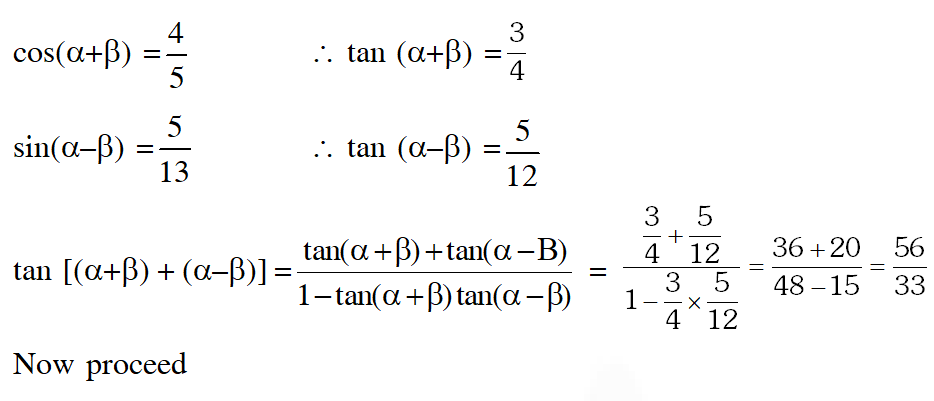Q. If $\mathrm{A}=\sin ^{2} \mathrm{x}+\cos ^{4} \mathrm{x},$ then for all real $\mathrm{x}:-$ (1) $1 \leq \mathrm{A} \leq 2$ (2) $\frac{3}{4} \leq \mathrm{A} \leq \frac{13}{16}$ (3) $\frac{3}{4} \leq \mathrm{A} \leq 1$ (4) $\frac{13}{16} \leq \mathrm{A} \leq 1$ [AIEEE-2011]
Ans. (3)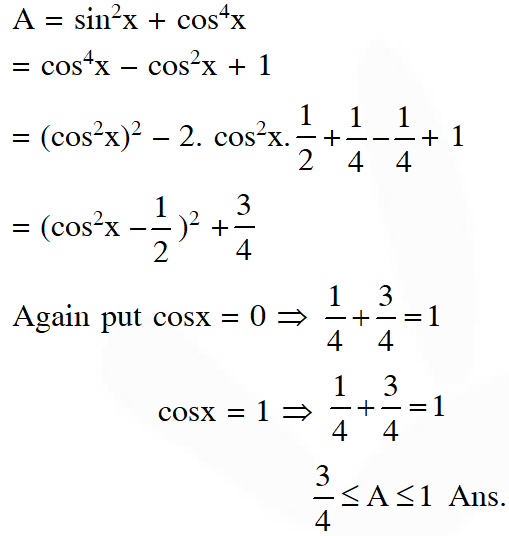Q. In a $\Delta \mathrm{PQR},$ if $3 \sin \mathrm{P}+4 \cos \mathrm{Q}=6$ and $4 \sin \mathrm{Q}+3 \cos \mathrm{P}=1,$ then the angle $\mathrm{R}$ is equal to: (1) $\frac{3 \pi}{4}$ (2) $\frac{5 \pi}{6}$ (3) $\frac{\pi}{6}$ (4) $\frac{\pi}{4}$ [AIEEE-2012]
Ans. (3)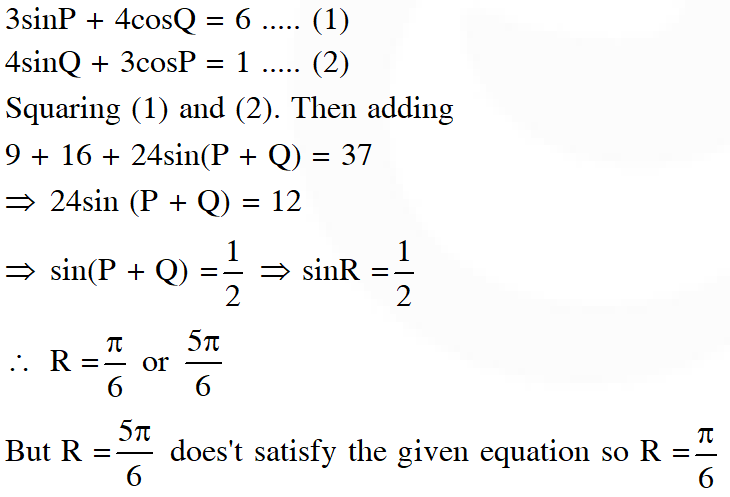Q. The expression $\frac{\tan \mathrm{A}}{1-\cot \mathrm{A}}+\frac{\cot \mathrm{A}}{1-\tan \mathrm{A}}$ can be written as (1) sinA cosA + 1 (2) secA cosecA + 1 (3) tanA + cotA (4) secA + cosecA [JEE-MAIN 2013]
Ans. (2)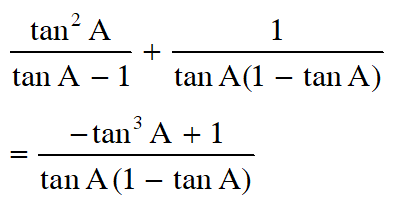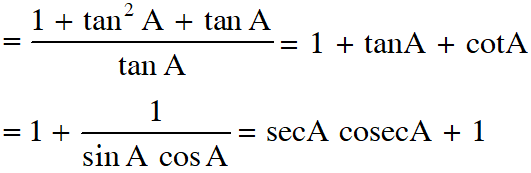Q. $\mathrm{ABCD}$ is a trapezium such that $\mathrm{AB}$ and $\mathrm{CD}$ are parallel and $\mathrm{BC} \perp \mathrm{CD} .$ If $\angle \mathrm{ADB}=\theta, \mathrm{BC}$ $=\mathrm{p}$ and $\mathrm{CD}=\mathrm{q},$ then $\mathrm{AB}$ is equal to (1) $\frac{\left(\mathrm{p}^{2}+\mathrm{q}^{2}\right) \sin \theta}{\mathrm{p} \cos \theta+\mathrm{q} \sin \theta}$ (2) $\frac{\mathrm{p}^{2}+\mathrm{q}^{2} \cos \theta}{\mathrm{p} \cos \theta+\mathrm{q} \sin \theta}$ (3) $\frac{\mathrm{p}^{2}+\mathrm{q}^{2}}{\mathrm{p}^{2} \cos \theta+\mathrm{q}^{2} \sin \theta}$ (4) $\frac{\left(p^{2}+q^{2}\right) \sin \theta}{(p \cos \theta+q \sin \theta)^{2}}$ [JEE-MAIN 2013]
Ans. (1)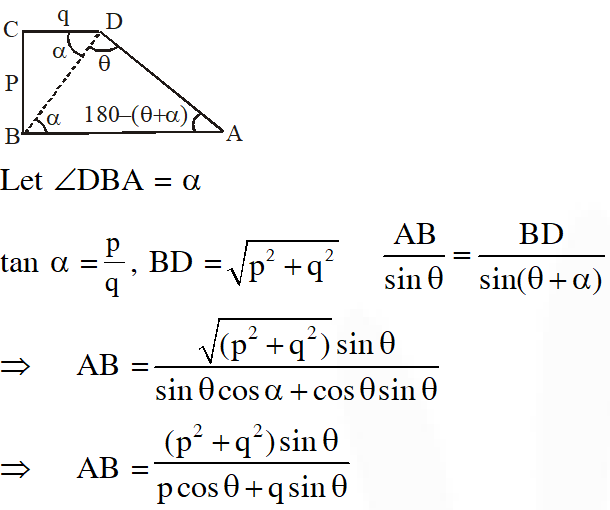Q. Let $\mathrm{f}_{\mathrm{K}}(\mathrm{x})=\frac{1}{\mathrm{k}}\left(\sin ^{\mathrm{k}} \mathrm{x}+\cos ^{\mathrm{k}} \mathrm{x}\right)$ where $\mathrm{x} \in \mathrm{R}$ and $\mathrm{k} \geq 1 .$ Then $\mathrm{f}_{4}(\mathrm{x})-\mathrm{f}_{6}(\mathrm{x})$ equals : (1) $\frac{1}{6}$ (2) $\frac{1}{3}$ (3) $\frac{1}{4}$ (4) $\frac{1}{12}$ [JEE-MAIN 2014]
Ans. (4)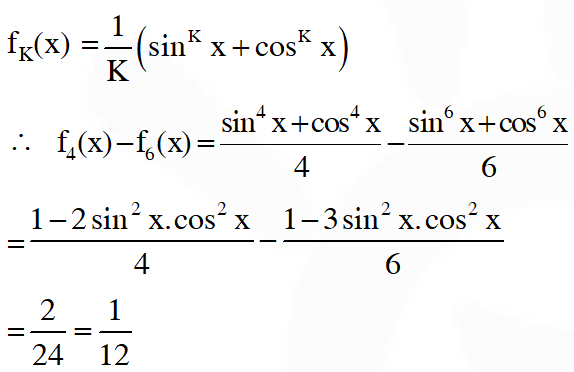Q. If $5\left(\tan ^{2} x-\cos ^{2} x\right)=2 \cos 2 x+9,$ then the value of $\cos 4 x$ is :- $(1)-\frac{7}{9}$ $(2)-\frac{3}{5}$ (3) $\frac{1}{3}$ (4) $\frac{2}{9}$ [JEE-MAIN 2017]
Ans. (1)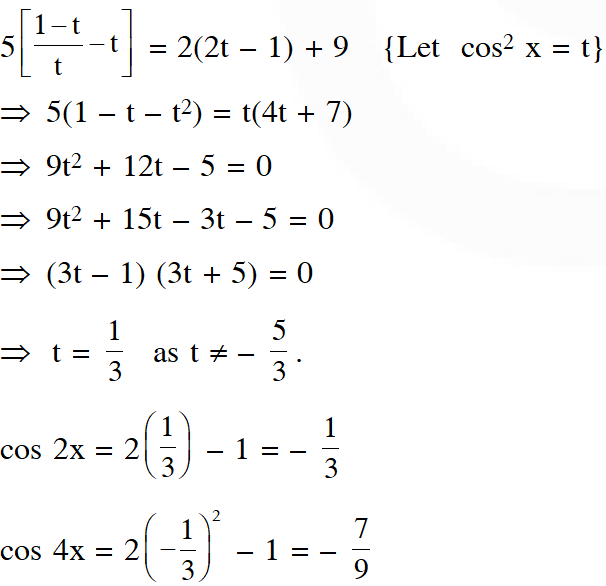zahid
Dec. 1, 2021, 1:05 p.m.
nice questions
Ftyyy
June 6, 2021, 10:11 a.m.
Fddtt
Sagnik Ghosh
Aug. 14, 2020, 12:37 p.m.
Thank you. These were very helpful.
Ganesh
July 3, 2020, 9:06 a.m.
Nice questions Make some more difficult Nice..
Cherry
June 20, 2020, 4:18 p.m.
You can have aa better explanation ....
Akhil
May 22, 2020, 7:38 a.m.
Chill bro
allu arjun's friend
April 7, 2020, 12:56 p.m.
nice perfomance from you i loved your work we students who are preparing for furture exams must need it a lot thank you so much
000
March 23, 2020, 12:40 p.m.
000000000